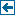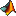Master index Index for chronux/spectral_analysis/continuous# Index for chronux/spectral_analysis/continuous

## Matlab files in this directory:CrossSpecMatc Multi-taper cross-spectral matrix - another routine, allows for multiple trials and channelscoherencyc Multi-taper coherency,cross-spectrum and individual spectra - continuous processcoherencyc_unequal_length_trials This routine computes the average multi-taper coherence for a given set of unequal length segments. It iscoherencysegc Multi-taper coherency, cross-spectrum and individual spectra with segmenting - continuous processcohgramc Multi-taper time-frequency coherence,cross-spectrum and individual spectra - continuous processescohmatrixc Multi-taper coherency,cross-spectral matrix - continuous processcreatedatamatc Helper function to create an event triggered matrix from univariateevoked Function to calculate the evoked response given continuous data in theextractdatac Extract segements of continuous data between t(1) and t(2)findpeaks Helper function to find peaks in a given continuous valued time series xfitlinesc fits significant sine waves to data (continuous data).ftestc computes the F-statistic for sine wave in locally-white noise (continuous data).locdetrend Remove running line fit (using local linear regression)-continuouslocsmooth Running line fit (using local linear regression) - 1d only, continuousmtdspecgramc Multi-taper derivative of the time-frequency spectrum - continuous processmtdspectrumc Multi-taper frequency derivative of the spectrum - continuous processmtfftc Multi-taper fourier transform - continuous datamtpowerandfstatc Multi-taper computation of the power and the fstatistic for a particular frequency - continuous processmtspecgramc Multi-taper time-frequency spectrum - continuous processmtspecgramtrigc Multi-taper event triggered time-frequency spectrum - continuous processmtspectrum_of_spectrumc Multi-taper segmented, second spectrum (spectrum of the log spectrum) for a continuous processmtspectrumc Multi-taper spectrum - continuous processmtspectrumc_unequal_length_trials This routine computes the multi-taper spectrum for a given set of unequal length segments. It ismtspectrumsegc Multi-taper segmented spectrum for a univariate continuous processmtspectrumtrigc Multi-taper event triggered time-frequency spectrum - continuous processnonst_stat Nonstationarity test - continuous processquadcof Helper function to calculate the nonstationary quadratic inverse matrixquadinv calculates the quadratic inverse eigenvectorsrmlinesc removes significant sine waves from data (continuous data).rmlinesmovingwinc fits significant sine waves to data (continuous data) using overlapping windows.runline Running line fit (local linear regression)spsvd Space frequency SVD of input data - continuous processes

Generated on Fri 28-Sep-2012 12:34:27 by m2html © 2005